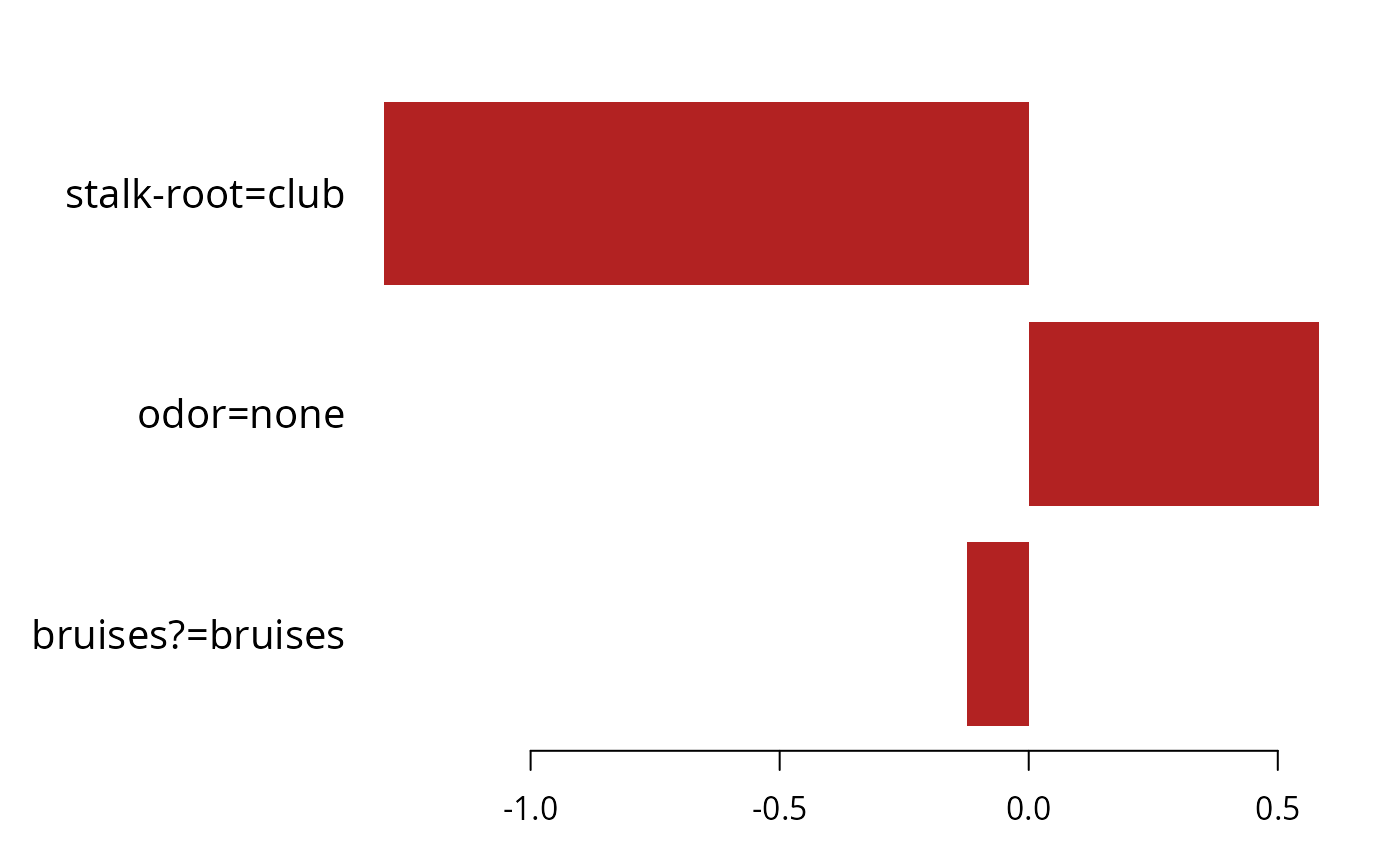Plot previously calculated feature contribution as a bar graph.

lgb.plot.interpretation(
tree_interpretation_dt,
top_n = 10L,
cols = 1L,
left_margin = 10L,
cex = NULL
)

## Arguments

tree_interpretation_dt a data.table returned by lgb.interprete. maximal number of top features to include into the plot. the column numbers of layout, will be used only for multiclass classification feature contribution. (base R barplot) allows to adjust the left margin size to fit feature names. (base R barplot) passed as cex.names parameter to barplot.

## Value

The lgb.plot.interpretation function creates a barplot.

## Details

The graph represents each feature as a horizontal bar of length proportional to the defined contribution of a feature. Features are shown ranked in a decreasing contribution order.

## Examples

# \donttest{
Logit <- function(x) {
log(x / (1.0 - x))
}
data(agaricus.train, package = "lightgbm")
labels <- agaricus.train$label dtrain <- lgb.Dataset( agaricus.train$data
, label = labels
)
set_field(
dataset = dtrain
, field_name = "init_score"
, data = rep(Logit(mean(labels)), length(labels))
)

data(agaricus.test, package = "lightgbm")

params <- list(
objective = "binary"
, learning_rate = 0.1
, max_depth = -1L
, min_data_in_leaf = 1L
, min_sum_hessian_in_leaf = 1.0
)
model <- lgb.train(
params = params
, data = dtrain
, nrounds = 5L
)
#> [LightGBM] [Info] Number of positive: 3140, number of negative: 3373
#> [LightGBM] [Warning] Auto-choosing row-wise multi-threading, the overhead of testing was 0.001044 seconds.
#> You can set force_row_wise=true to remove the overhead.
#> And if memory is not enough, you can set force_col_wise=true.
#> [LightGBM] [Info] Total Bins 232
#> [LightGBM] [Info] Number of data points in the train set: 6513, number of used features: 116
#> [LightGBM] [Warning] No further splits with positive gain, best gain: -inf
#> [LightGBM] [Warning] No further splits with positive gain, best gain: -inf
#> [LightGBM] [Warning] No further splits with positive gain, best gain: -inf
#> [LightGBM] [Warning] No further splits with positive gain, best gain: -inf
#> [LightGBM] [Warning] No further splits with positive gain, best gain: -inf

tree_interpretation <- lgb.interprete(
model = model
, data = agaricus.test\$data
, idxset = 1L:5L
)
lgb.plot.interpretation(
tree_interpretation_dt = tree_interpretation[[1L]]
, top_n = 3L
)# }